Cisco CCENT IP Addressing & Subnetting Part I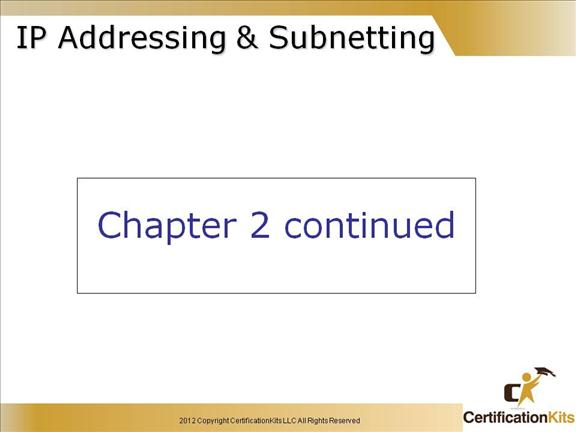One of the most important topics in any discussion of TCP/IP is IP addressing. An IP address is a numeric identifier assigned to each machine on an IP network. It designates the specific location of a device on the network.

An IP address is a software address, not a hardware address. IP addressing was designed to allow a host on one network to communicate with a host on a different network, regardless of the type of LANs the hosts are participating in.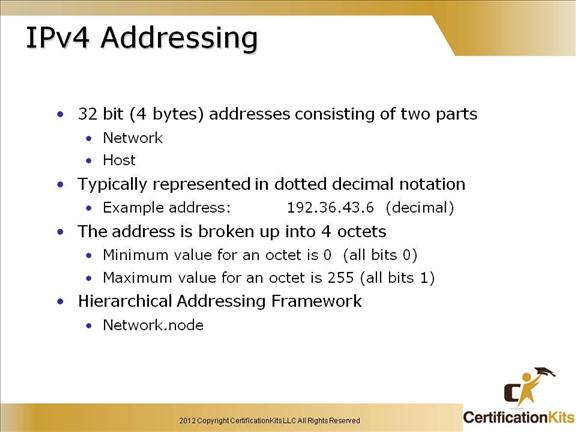Before we get into the more complicated aspects of IP addressing, you need to understand some of the basics:

Bit = 1 digit (either a one or a zero)

Byte = 7 or 8 bits (depends on parity)  From an IP address perspective, assume 8.

Octet = Always 8 bits

IPv4 addresses are 32 bit (4 byte) addresses consisting of two parts, a network portion and a host portion.  It is typically represented in dotted decimal notation.  An example is 192.36.43.6.  Each octet has a value between 0 and 255 where 0 is all bits being 0 and 255 is all bits being 1.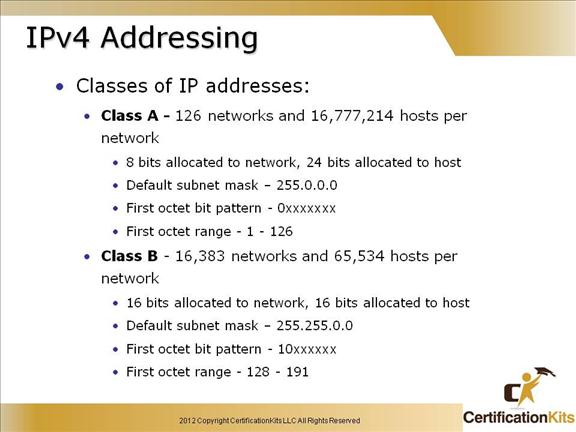Defines Class A and Class B IP address characteristics.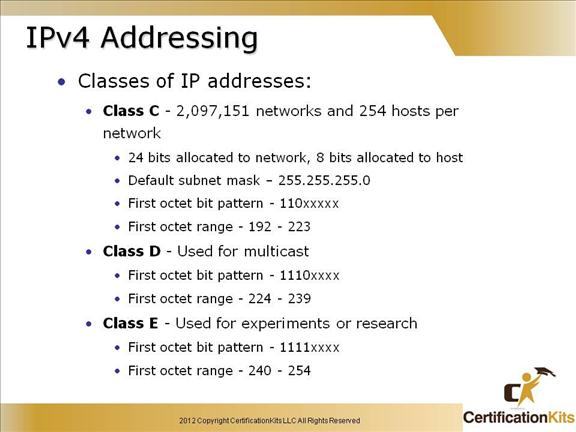Defines Class C, Class D and Class E IP address characteristics.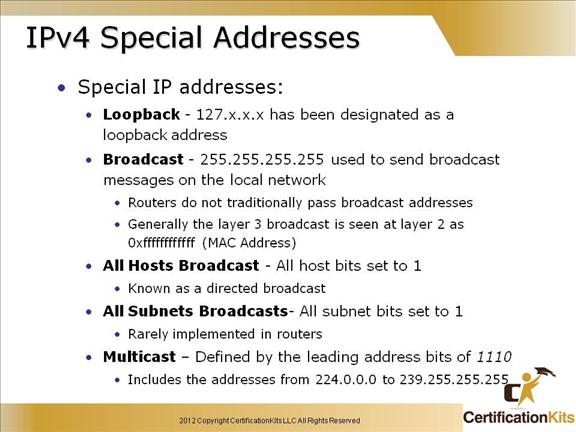If an IP device wants to communicate with all devices on the local network, it sets the destination address to all 1s (255.255.255.255) and transmits the packet. For example, hosts that do not know their network number and are asking some server for it may use this address. The local broadcast is never routed.

A local loopback address is used to let the system send a message to itself for testing. A typical local loopback IP address is 127.0.0.1.

When neither a statically nor a dynamically configured IP address is found on startup, those hosts supporting IPv4 link-local addresses (RFC 3927) will generate an address in the 169.254/16 prefix range. This address can be used only for local network connectivity and operates with many caveats, one of which is that it will not be routed. You will mostly see this address as a failure condition when a PC fails to obtain an IP address.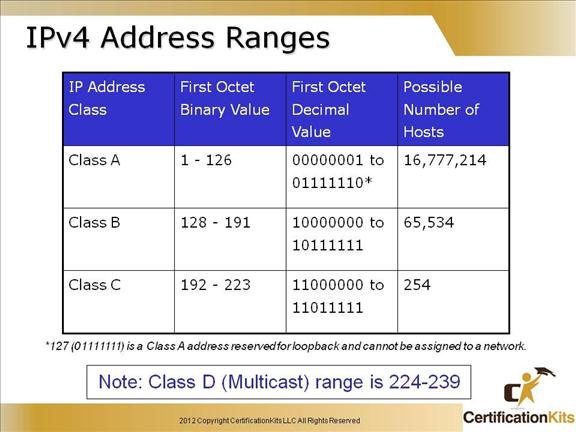The number of possible hosts is a Class A address are much greater than the number of possible hosts in a Class C address.  Fortunately with the use of Variable Length Subnet Masks (VLSM), classfull boundaries can be removed to make better use of address space.  In order to properly route IP packets utilizing VLSM, a classless routing protocol like OSPF, EIGRP or RIP version 2 must be used.  Routing protocols such as RIP version 1 or IGRP do not recognize VLSM as they are classful routing protocols.

The designers of the IP address scheme said that the first bit of the first byte in a Class A network address must always be off, or 0. This means a Class A address must be between 0 and 126 (127 is reserved for loopback address).

In a Class B network, the RFCs state that the first bit of the first byte must always be turned on, but the second bit must always be turned off. If you turn the other six bits all off and then all on, you will find the range for a Class B network:

10000000 = 128

10111111 = 191

For Class C networks, the RFCs define the first two bits of the first octet always turned on, but the third bit can never be on. Following the same process as the previous classes, convert from binary to decimal to find the range. Here’s the range for a Class C network:

11000000 = 192

11011111 = 223

So, if you see an IP address that starts at 192 and goes to 223, you’ll know it is a Class C IP address.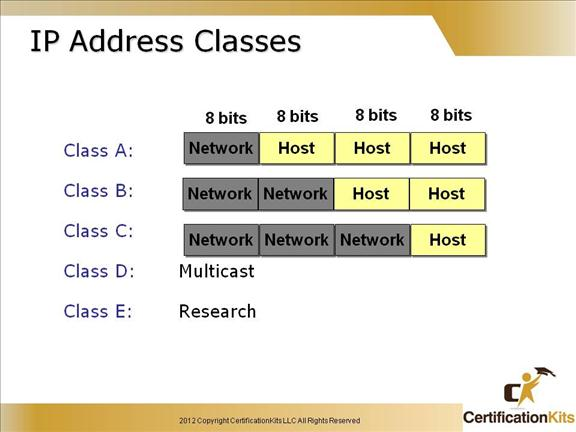The designers of the Internet decided to create classes of networks based on network size. For the small number of networks possessing a very large number of nodes, they created the rank Class A network.

At the other extreme is the Class C network, which is reserved for the numerous networks with a small number of nodes.

The class distinction for networks between very large and very small is predictably called the Class B network.

Subdividing an IP address into a network and node address is determined by the class designation of one’s network.

With the advent of Variable Length Subnet Masks (VLSM), the distinction between the different classes of IP addresses are not as important as they used to be.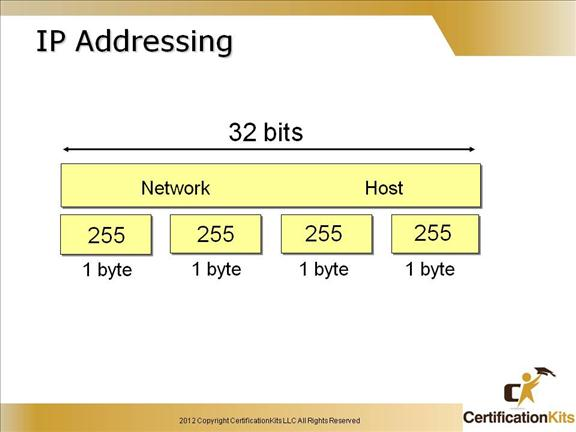An IP address consists of 32 bits of information.  These bits are divided into four sections, referred to as octets or bytes, each containing 1 byte (8 bits).   The address is logically separated into a network portion and a host portion.  The subnet mask defines where the network portion ends and the host portion begins.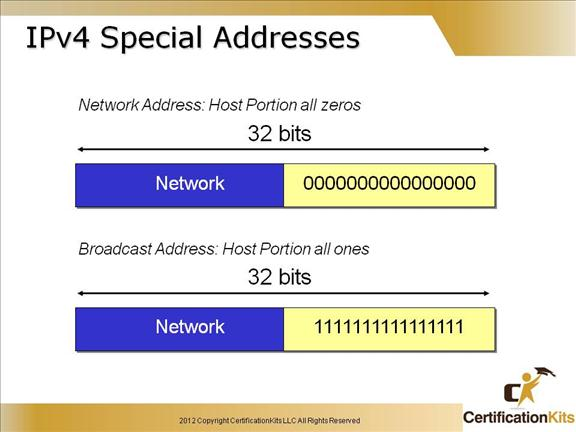An IP address that has binary 0s in all host bit positions is reserved for the network address. Therefore, as a Class A network example, 10.0.0.0 is the IP address of the network containing the host 10.1.2.3. As a Class B network example, the IP address 172.16.0.0 is a network address, while 192.16.1.0 would be a Class C network. A router uses the network IP address when it searches its IP route table for the destination network location.

The decimal numbers that fill the first two octets in a Class B network address are assigned. The last two octets contain 0s because those 16 bits are for host numbers and are used for devices that are attached to the network. In the IP address 172.16.0.0, the first two octets are reserved for the network address; it is never used as an address for any device that is attached to it. An example of an IP address for a device on the 172.16.0.0 network would be 172.16.16.1. In this example, 172.16 is the network address portion and 16.1 is the host address portion.

To send data to all the devices on a network, a broadcast address is used. Broadcast IP addresses end with binary 1s in the entire host part of the address (the host field).

For the network in the example (172.16.0.0), in which the last 16 bits make up the host field (or host part of the address), the broadcast that would be sent out to all devices on that network would include a destination address of 172.16.255.255.

The directed broadcast is capable of being routed. However, for some versions of the Cisco IOS operating system, routing directed broadcasts is not the default behavior.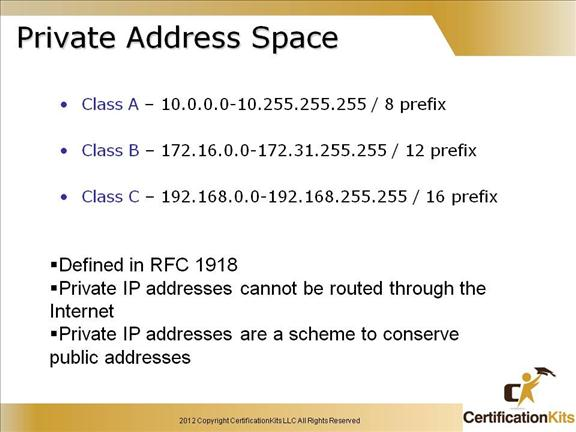The people who sat around and created the IP addressing scheme also created what we call private IP addresses.

These addresses can be used on a private network, but they’re not routable through the Internet. This is designed for the purpose of creating a measure of well-needed security, but it also conveniently saves valuable IP address space.

Again, now shown in binary:

Class A: 00001010

Class B: 10101100.00010000 through 10101100.00011111

Class C: 11000000.10101000

Cisco CCENT Private IP Question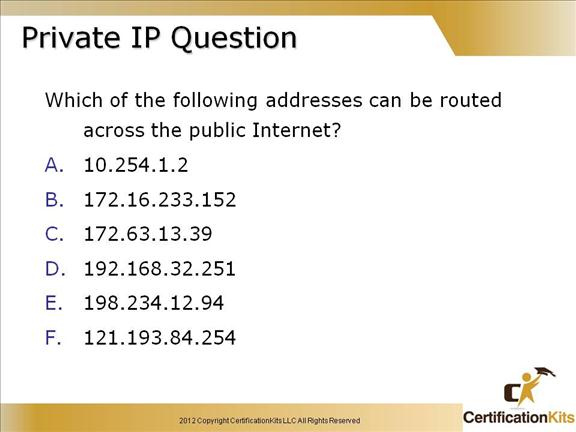The following addresses can be routed across the public Internet:

C. 172.63.13.39

E. 198.234.12.94

F. 121.193.84.254

The following addresses fall under RFC 1918 and are not routed across the public Internet:

A. 10.254.1.2

B. 172.16.233.152

D. 192.162.32.251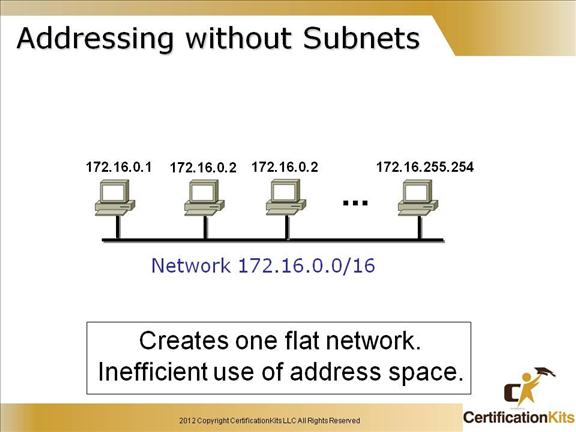Without creating subnetworks, all hosts would be on one large network. Not good…really not good.  This type of network creates one large broadcast domain.  It is not scalable.  Routers are used to break up broadcast domains and allow for communication between different ip subnets.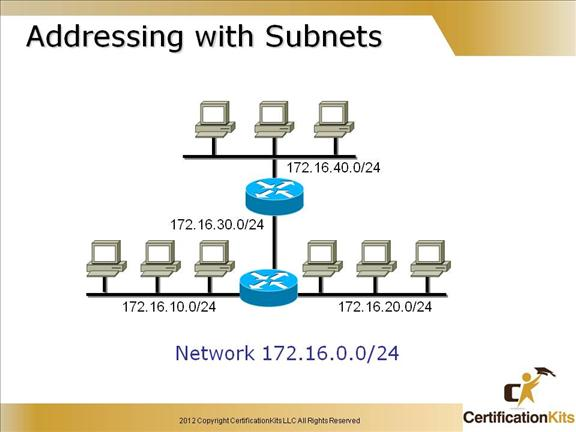There are loads of reasons in favor of subnetting. Some of the benefits include:

Reduced network traffic

Optimized network performance

Simplified management

Facilitated spanning of large geographical distances

Cisco CCENT How do you determine the mask to use?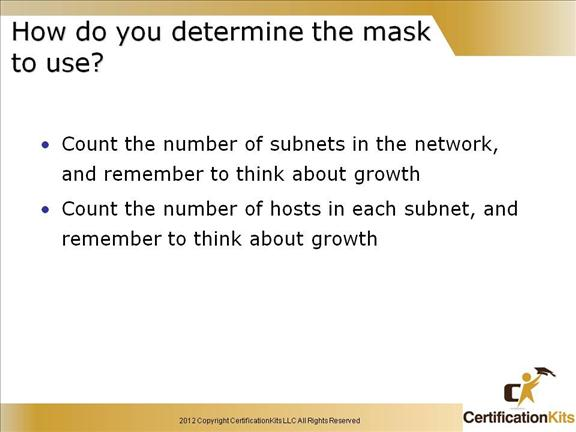1. Determine the number of required network IDs:

One for each subnet

One for each wide area network connection

2. Determine the number of required host IDs per subnet:

One for each TCP/IP host

One for each router interface

For example if you are provided a class C address and need to carve it up and are given requirements that you need one subnet to support 120 hosts, one subnet to support 50 hosts and four subnets to support 10 host each you can carve it up as follows:

Cisco CCENT After you Choose a Possible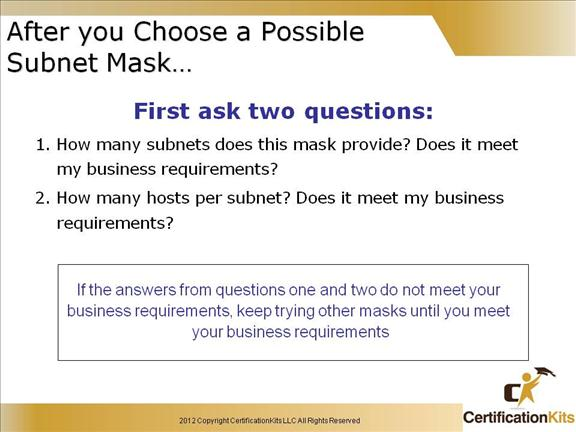This slide shows how to determine if a certain subnet mask will meet your business requirements of your Internetwork. It lists questions to ask when determining how to allocate IP addresses and subnets.  Remember to account for growth.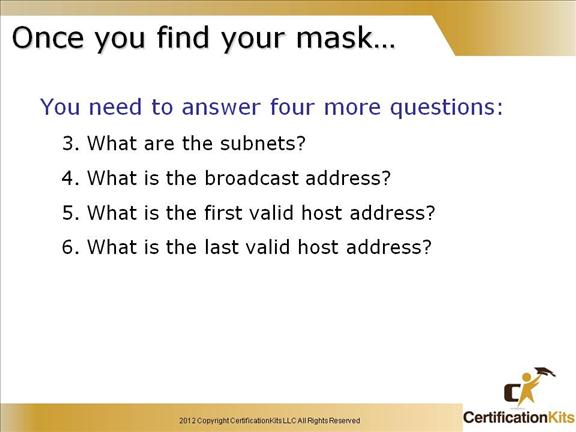Cisco CCENT Now, here is how to get Six Answers!This slide shows you how to achieve the answers to the six important subnetting questions:

How many subnets? 2x = number of subnets. x is the number of masked bits, or the 1s. For example, in 11000000, the number of ones gives us 22 subnets. In this example, there are 4 subnets.

How many hosts per subnet? 2x – 2 = number of hosts per subnet. x is the number of unmasked bits, or the 0s. For example, in 11000000, the number of zeros gives us 26 – 2 hosts. In this example, there are 62 hosts per subnet.

What are the valid subnets? 256 – subnet mask = block size, or base number. For example, 256 – 192 = 64. 64 is the first subnet. The next subnet would be the base number itself, or 64 + 64 = 128, (the second subnet).

You keep adding the base number to itself until you reach the value of the subnet mask, which is not a valid subnet because all subnet bits would be turned on (1s).

Whats the broadcast address for each subnet? The broadcast address is all host bits turned on, which is the number immediately preceding the next subnet.

What are the valid hosts? Valid hosts are the numbers between the subnets, minus all 0s and all 1s.

Cisco CCENT Classless Inter-Domain Routing
(CIDR)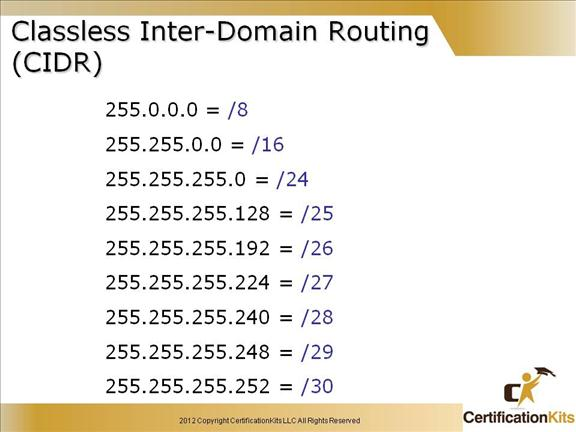Another term you need to familiarize your self with is Classless Inter-Domain Routing (CIDR).

It is really just the method that ISP’s (Internet Service Providers) use to allocate an amount of addresses to a company, home—a customer.

They provide addresses in a certain block size—something we will be going into in greater detail later in this chapter.

So when you receive a block of addresses from an ISP, what you’ll get will look something like this: 192.168.10.32/28. What this is telling you is what your subnet mask is.

The slash notation (/) means how many bits are turned on (1’s). Obviously, the maximum could only be /32 because a byte is 8 bits and there are four bytes in an IP address: (4×8=32).   In the example, 192.168.10.32/28 means the address range provided including subnet and broadcast is 192.168.10.32 – 192.168.10.47.

But keep in mind that the largest subnet mask available (regardless of the class of address) can only be a /30 because you’ve got to keep at least two bits for host bits.

Cisco CCENT IP Subnet-Zero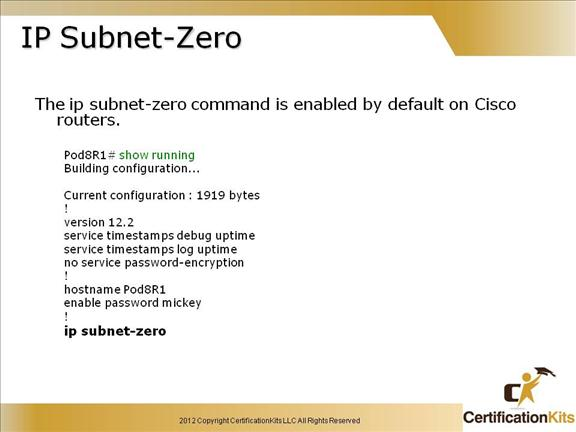The ip subnet-zero command provides the ability to configure and route to subnet 0 subnets.

Subnetting with a subnet address of 0 is discouraged because of the confusion inherent in having a network and a subnet with indistinguishable addresses. It has a major benefit in that it utilizes address space more efficiently.

Cisco CCENT IPv4 Subnet Calculation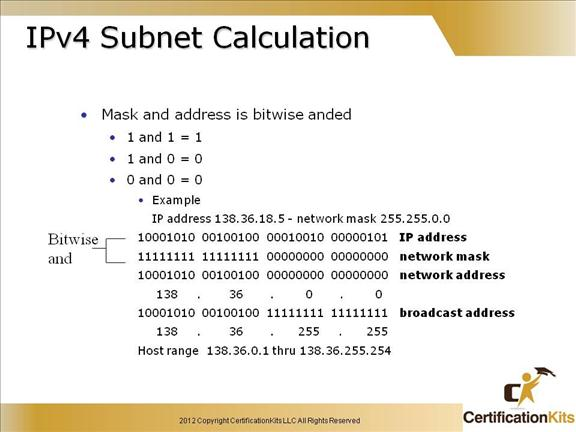The slide indicates one way to determine both the network address given an address and mask utilizing a logical AND.  The network address is all 0’s in the host portion of the address and the broadcast is all 1’s in the host portion of the address.

Cisco CCENT Binary to Decimal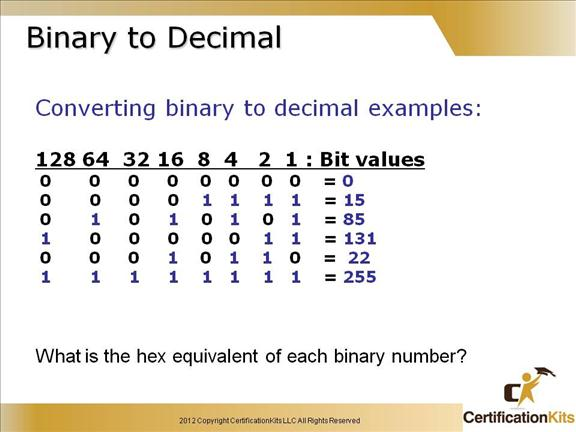We discussed this in chapter 1, but it is important enough to review at this point:

It’s pretty simple really. The digits used are limited to either a 1(one) or a 0 (zero), with each digit being called one bit.  Typically, you count either four or eight bits together, with these being referred to as a nibble or a byte, respectively.

What interests us in binary numbering is the value represented in a decimal format—the typical decimal format being our base ten number scheme we’ve all used since kindergarten.

The binary numbers are placed in a value spot; starting at the right and moving left, with each spot having double the value of the previous spot.

128  64  32  16  8  4  2  1

So explaining a couple of examples on the slide are as follows:

85 equals 01010101 which equates to 64 + 16 + 4 + 1 = 85

131 equals 10000011 which equates to 128 + 2 + 1 = 131 simple really.

Cisco CCENT Binary (Cont.)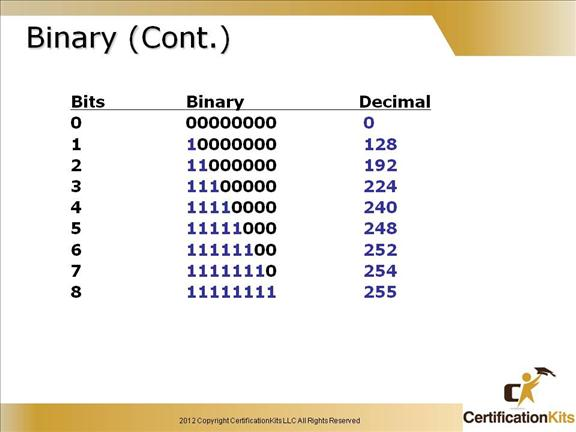Here is a binary chart that is best just to memorize.  You will need to know this off the top of your head as we delve deeper into subnetting and when you take the CCNA test.  Notice that it is no more than taking the information just learned on the previous slide and performing a little addition.  Remember the bit values were as follows:

128  64  32  16  8  4  2  1

So 11000000 equates to 128 + 64 = 192

11100000 equates to 128 + 64 + 32 = 224

11110000 equates to 128 + 64 + 32 + 16 = 240

11111000 equates to 128 + 64 + 32 + 16 + 8 = 248

11111100 equates to 128 + 64 + 32 + 16 + 8 + 4 = 252

11111110 equates to 128 + 64 + 32 + 16 + 8 + 4 + 2 = 254

11111111 equates to 128 + 64 + 32 + 16 + 8 + 4 + 2 + 1 = 255